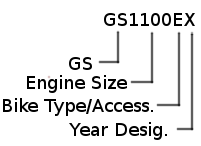## GS Model Information Chart

The GS Model Information Chart is a way of determining what the full model designation is of your motorcycle. This info is useful when purchasing insurance or when buying replacement parts. The model designation is similar to a VIN number in that it breaks down to describe multiple items, such as the year it was made, the style of the mortorcycle, as well as some of the features of the bike.

Here is how a model designation breaks down.GS = that's pretty self-explanatory
Engine Size = cc's of your engine
Bike Type/Assess. = Bike type or accessories that came on the bike. They are
'X'=basic model, 'E'=sport model, 'G'=shaft drive, 'L'=cruiser, 'K'=fairing & hardbags, 'S'=sport or Katana, 'M'=Katana, 'A'=automatic transmission
Year Desig. = Year the bike was built

### Use this chart to determine the full model designation for your motorcycle

Simply scroll down the chart to find the model of your bike. We will use a 1981 GS1100EX listed above as an example. In looking at the graphic, we see that it is a 1100cc GS, in a sport model. Follow along horizontally in the chart and you should see an X for the year of the bike. The year 1981 has a 'X" for a year designation so the complete model identifier for your bike would be GS1100EX.

It should be noted that sometimes you won't find your bike listed in a particular year or the model may be completely missing from the chart. This happens often with non-US bikes. If your model is missing or the year isn't marked, then contact Frank and let him know so that the database can be updated.

Sometimes even with all this, it can be difficult to tell what your full model designation is. Frank has the "Suzuki Motorcycle and ATV Indentification Guide, 1970-1990". Send an email to Frank including your engine number, VIN and color of your bike and he should be able to identify it for you. Again, this is only for US marketed bikes.

#### Year Designation

76(A) 77(B) 78(C) 79(N) 80(T) 81(X) 82(Z) 83(D) 84(E) 85(F)
GS125 X X X X X X X
GS250T X X
GS300L X X X
GS400 X X X X X X X X
GS400E X X X X X X
GS400L X X X X
GS400S X X
GS400X X X
GS425 X
GS425E X
GS425L X
GS450A X X
GS450E X X X X X X
GS450GA X X X
GS450L X X X X X X
GS450S X X X X X X
GS450T X X X X
GS450TX X X
GS500E X X X X X X X X X
GS550 X X X
GS550E X X X X X X X X
GS550ES X X X
GS550ES3 X
GS550L X X X X X X X
GS550M X X X
GS550T X
GS650E X X X
GS650G X X X
GS650GL X X X
GS650GM X X X X X
GS650GT X X X X X
GS650L X X X
GS650M X X X
GS700E X
GS700ES X
GS750 X X X X
GS750D X X
GS750E X X X X X X X X X
GS750EF X X X
GS750ES X X X
GS750G X X
GS750GL X
GS750L X X X X
GS750S X X X
GS750T X X
GS850G X X X X X X X X
GS850GL X X X X X
GS1000 X X X X
GS1000E X X X
GS1000G X X X X
GS1000GL X X X
GS1000H X X X
GS1000L X X X
GS1000S X X X X
GS1100E X X X X
GS1100ES X
GS1100G X X X X X
GS1100GK X X X X X
GS1100GK2 X
GS1100GL X X
GS1100L X X X X
GS1100S X X X
GS1150EF X X
GS1150ES X X
GS1150ES3 X
GSX250E X
GSX400F X X X X X
GSX750E X X X X
GSX750ES X X X
GSX1100E X X X X
GSX1100EF X
GSX1100ES X X X
GSX1100ES3 X
GSX1100S X X X X